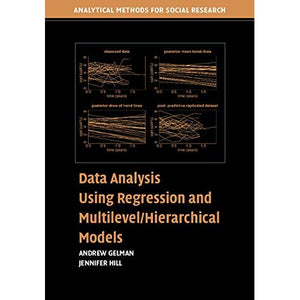># Data Analysis Using Regression and Multilevel/Hierarchical Models (Analytical Methods for Social Research)

• £26.69
• Save £13

Andrew Gelman
Cambridge University Press, 6/14/2007
EAN 9780521686891, ISBN10: 052168689X

Paperback, 648 pages, 25.4 x 17.9 x 3.5 cm
Language: English

Data Analysis Using Regression and Multilevel/Hierarchical Models, first published in 2007, is a comprehensive manual for the applied researcher who wants to perform data analysis using linear and nonlinear regression and multilevel models. The book introduces a wide variety of models, whilst at the same time instructing the reader in how to fit these models using available software packages. The book illustrates the concepts by working through scores of real data examples that have arisen from the authors' own applied research, with programming codes provided for each one. Topics covered include causal inference, including regression, poststratification, matching, regression discontinuity, and instrumental variables, as well as multilevel logistic regression and missing-data imputation. Practical tips regarding building, fitting, and understanding are provided throughout.

1. Why?
2. Concepts and methods from basic probability and statistics
Part I. A. Single-Level Regression
3. Linear regression
the basics
4. Linear regression
before and after fitting the model
5. Logistic regression
6. Generalized linear models
Part I. B. Working with Regression Inferences
7. Simulation of probability models and statistical inferences
8. Simulation for checking statistical procedures and model fits
9. Causal inference using regression on the treatment variable
10. Causal inference using more advanced models
Part II. A. Multilevel Regression
11. Multilevel structures
12. Multilevel linear models
the basics
13. Multilevel linear models
varying slopes, non-nested models and other complexities
14. Multilevel logistic regression
15. Multilevel generalized linear models
Part II. B. Fitting Multilevel Models
16. Multilevel modeling in bugs and R
the basics
17. Fitting multilevel linear and generalized linear models in bugs and R
18. Likelihood and Bayesian inference and computation
19. Debugging and speeding convergence
Part III. From Data Collection to Model Understanding to Model Checking
20. Sample size and power calculations
21. Understanding and summarizing the fitted models
22. Analysis of variance
23. Causal inference using multilevel models
24. Model checking and comparison
25. Missing data imputation
Appendixes
A. Six quick tips to improve your regression modeling
B. Statistical graphics for research and presentation
C. Software
References.

'Data Analysis Using Regression and Multilevel/Hierarchical Models' ... careful yet mathematically accessible style is generously illustrated with examples and graphical displays, making it ideal for either classroom use or self-study. It appears destined to adorn the shelves of a great many applied statisticians and social scientists for years to come.' Brad Carlin, University of Minnesota